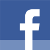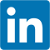# Randomizing a CSV File with Standard C++

published at 01.11.2016 20:29 by Jens Weller

For this years student program I had to come up with a way to randomly select n students from all applicants. I wanted to do this in a clean and nice C++ program. So here it is:

```int main(int argc, char *argv[])
{
std::string path("./input.csv");
if(argc > 1)
path = argv;
std::vector vec;
std::string line;
std::ifstream in(path);
while(std::getline(in,line))
vec.push_back(line);
if(vec.size() < 2)
return -1;
//don't randomize the header line (should not contain any @, every line has an email other wise, hence data always has an @)
auto beg = vec.begin();
if(beg->find("@") == std::string::npos)
beg++;
std::random_device rd;
std::mt19937 g(rd());
std::shuffle(beg,vec.end(),g);

std::ofstream out("random.csv");
auto it = vec.begin();
char del = ';';
if(it->find(',') != std::string::npos)
del = ',';
out << *it++ << del << "Index\n";
int i = 0;
std::for_each(it,vec.end(),[&out,del,&i](const std::string& line){out << line << del << ++i<< "\n";});
std::cout << "randomizer finished";
return 0;
}```

Quick walk through: I load the whole csv file (actually a mysql table dump) into a vector, where each line is an entry. If there is only one entry, we are done. Next I'd like to know if there is an '@' in the first line. I don't expect the header to contain this, but as every student registered with an email, its a handy way to prevent that the header is ending up in the data.

With C++11 came <random>, and it contains everything I need. As random_shuffle is deprecated, I have to use shuffle and provide an RNG. I chose the mersenne twister, initialized with std::random_device. After the vector is shuffled, I write the result to random.csv. std::copy would be very good to do this easily, but I want to add an index to the data. This is simply to make the notification easy, as with this year its 38 students, I simply can create a conditional for the mailing on index < 38 to either state you're accepted or not. In order for this to work, I have to figure out if the delimeter is , or ;, and then add the index. Also I have to add the name of this field to the header.

The program was compiled with the Visual C++ build tools, as my usual MinGW installation from Qt does not provide a proper <random> implementation under windows. All students were notified today.

Join the Meeting C++ patreon community!
This and other posts on Meeting C++ are enabled by my supporters on patreon!